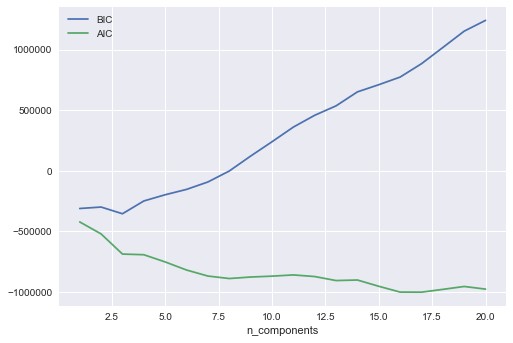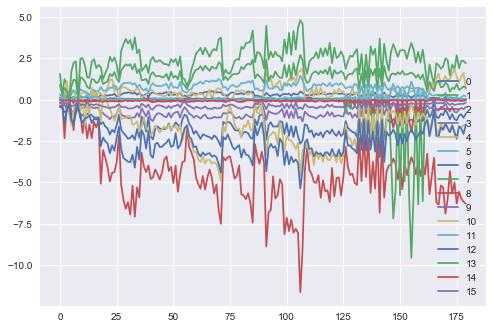# Time Series Clustering - Mixture Models for Clustering

Published:

Time Series Clustering - Mixture Models for Clustering

Following are the steps in using mixture models for Clustering:-

1. Fit the mixture model
2. Compute

represents the posterior probability that point i belongs to cluster k.

Previous procedure is soft clustering.

Assuming this is small, it may be reasonable to compute a hard clustering using the MAP estimte, given by

We call this procedure

import os
import pandas as pd
%matplotlib inline
import matplotlib.pyplot as plt
import seaborn as sns; sns.set()
import numpy as np
from sklearn.mixture import GaussianMixture

path = '/Users/avinashbarnwal/Desktop/Machine Learning/Daily'
os.chdir(path)


One of the most important parameter is covariance type which is “full” leading to exhaustive search

n_components = np.arange(1, 21)
models = [GaussianMixture(n, covariance_type='full', random_state=0).fit(X)
for n in n_components]

plt.plot(n_components, [m.bic(X) for m in models], label='BIC')
plt.plot(n_components, [m.aic(X) for m in models], label='AIC')
plt.legend(loc='best')
plt.xlabel('n_components')gmm = GaussianMixture(n_components=16, covariance_type='full').fit(X)
for i in range(16):
plt.plot(gmm.means_[i], label=i)
plt.legend(loc='best')